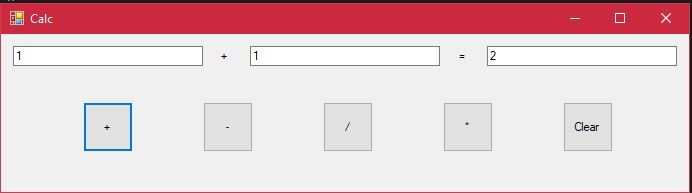# How to Create a Simple Calculator in Visual Studio 2017 – Part II

We have created  a simple calculator in the first part of this tutorial. In this part we will see how the code works and then try to improve some functionalities of it.

# Code Explanation

```using System;
using System.Collections.Generic;
using System.ComponentModel;
using System.Data;
using System.Drawing;
using System.Linq;
using System.Text;
using System.Threading.Tasks;
using System.Windows.Forms;

namespace SimpleCalc
{
public partial class frmCalc : Form
{
double dblNumber1 = 0;
double dblNumber2=0;
double dblAnswer = 0;
public frmCalc()
{
InitializeComponent();
}

private void btnAdd_Click(object sender, EventArgs e)
{
dblNumber1 = Double.Parse(txtNumber1.Text);
dblNumber2 = Double.Parse(txtNumber2.Text);
dblAnswer = dblNumber1 + dblNumber2;
txtAnswer.Text = dblAnswer.ToString();
}

private void btnSub_Click(object sender, EventArgs e)
{
dblNumber1 = Double.Parse(txtNumber1.Text);
dblNumber2 = Double.Parse(txtNumber2.Text);
dblAnswer = dblNumber1 - dblNumber2;
txtAnswer.Text = dblAnswer.ToString();
}

private void btnDiv_Click(object sender, EventArgs e)
{
dblNumber1 = Double.Parse(txtNumber1.Text);
dblNumber2 = Double.Parse(txtNumber2.Text);
dblAnswer = dblNumber1 / dblNumber2;
txtAnswer.Text = dblAnswer.ToString();
}

private void btnMulti_Click(object sender, EventArgs e)
{
dblNumber1 = Double.Parse(txtNumber1.Text);
dblNumber2 = Double.Parse(txtNumber2.Text);
dblAnswer = dblNumber1 * dblNumber2;
txtAnswer.Text = dblAnswer.ToString();
}

private void btnClear_Click(object sender, EventArgs e)
{
double dblNumber1 = 0;
double dblNumber2 = 0;
double dblAnswer = 0;
txtNumber1.Text = "";
txtNumber2.Text = "";
txtAnswer.Text = "";
}
}
}
```

## Variable declaration and initializing

```double dblNumber1 = 0;
double dblNumber2=0;
double dblAnswer = 0;```

Here we are declaring 3 variables of the double type and with value 0 assign for them.

## Add button click event

```private void btnAdd_Click(object sender, EventArgs e)
{
dblNumber1 = Double.Parse(txtNumber1.Text);
dblNumber2 = Double.Parse(txtNumber2.Text);
dblAnswer = dblNumber1 + dblNumber2;
txtAnswer.Text = dblAnswer.ToString();
}```

This is the part of the code which will run when you click the Add Button (btnAdd) .

### Double.Parse() Method

This method is used to convert a string that contain a number to the equivalent double-precision floating-point number. You can read more about this method here .

In this example we are taking the value of the Text property of the button (which is a string) and converting it into a double using the Double.Parse method. We are storing this double value in variables dblNumber1 and dblNumber2 which correspond to numbers entered  to txtNumber1 and txtNumber2. (Code line 3 & 4)

Then we add values of those variables and store in the variable dblAnswer. (Code line 5).

Now as we have calculated the answer we have to display it in the txtAnswer TextBox. We can do this by assigning value of dblAnswer to Text property of txtAnswer. But to do that we have to first convert double into a string.

### ToString() Method

This method is used to convert a number to its string representation. You can read more about this method here

We use this method to convert the calculated answer to a string and assign it to the Text property of txtAnswer. (Code line 6)

## Subtract button click event

```private void btnSub_Click(object sender, EventArgs e)
{
dblNumber1 = Double.Parse(txtNumber1.Text);
dblNumber2 = Double.Parse(txtNumber2.Text);
dblAnswer = dblNumber1 - dblNumber2;
txtAnswer.Text = dblAnswer.ToString();
}```

Functionality of the above code is similar to that of Add button event. Only difference is when calculating the answer we subtract dblNumber2 from dblNumber1 and assign it to dblAnswer (Code line 6).

## Divide button and Multiply button click events

```private void btnDiv_Click(object sender, EventArgs e)
{
dblNumber1 = Double.Parse(txtNumber1.Text);
dblNumber2 = Double.Parse(txtNumber2.Text);
dblAnswer = dblNumber1 / dblNumber2;
txtAnswer.Text = dblAnswer.ToString();
}```
```private void btnMulti_Click(object sender, EventArgs e)
{
dblNumber1 = Double.Parse(txtNumber1.Text);
dblNumber2 = Double.Parse(txtNumber2.Text);
dblAnswer = dblNumber1 * dblNumber2;
txtAnswer.Text = dblAnswer.ToString();
}```

Same as above but dblNumber1 divide by dblNumber2 and dblNumber1 multiply by dblNumber2 assigned to dblAnswer in each case.

## Clear button click event

```private void btnClear_Click(object sender, EventArgs e)
{
double dblNumber1 = 0;
double dblNumber2 = 0;
double dblAnswer = 0;
txtNumber1.Text = "";
txtNumber2.Text = "";
txtAnswer.Text = "";
}```

When you click this button you rest everything. Value of the all the variables are set to 0 and Text property of all TextBoxes set to “” which is an empty string to clear the already entered values.

# ImprovementsYou might have notice that the label lblOperation have a question mark on it. Let’s change the code so that it will change to match the operation when an operation carried out .

`lblOperation.Text = "+";`

Add the above code to btnAdd click event. Now the code should look this.

```private void btnAdd_Click(object sender, EventArgs e)
{
dblNumber1 = Double.Parse(txtNumber1.Text);
dblNumber2 = Double.Parse(txtNumber2.Text);
dblAnswer = dblNumber1 + dblNumber2;
lblOperation.Text = "+";
txtAnswer.Text = dblAnswer.ToString();
}```

If you run the code and do an “Add” operation, Text of the lblOperation will change to “+”.Let’s change the code to show the operation as well.

Add following code to btnSub , btnDiv , btnMulti respectively.

`lblOperation.Text = "-";`
`lblOperation.Text = "/";`
`lblOperation.Text = "*";`

But when you click the Clear button Text of lblOperation doesn’t change to question mark yet.Let’s add the following code to click event of btnClear to solve this.

`lblOperation.Text = "?";`

Now when you click the Clear button it will reset to the initial state.

In the next part of this tutorial we will learn to prevent errors such as entering non numeric inputs and about using functions to prevent repetition of codes.#### Kavinda

Proud UCSCian | Proud Devan | Computer Geek | Superman | Love Chess & Programming

This site uses Akismet to reduce spam. Learn how your comment data is processed.